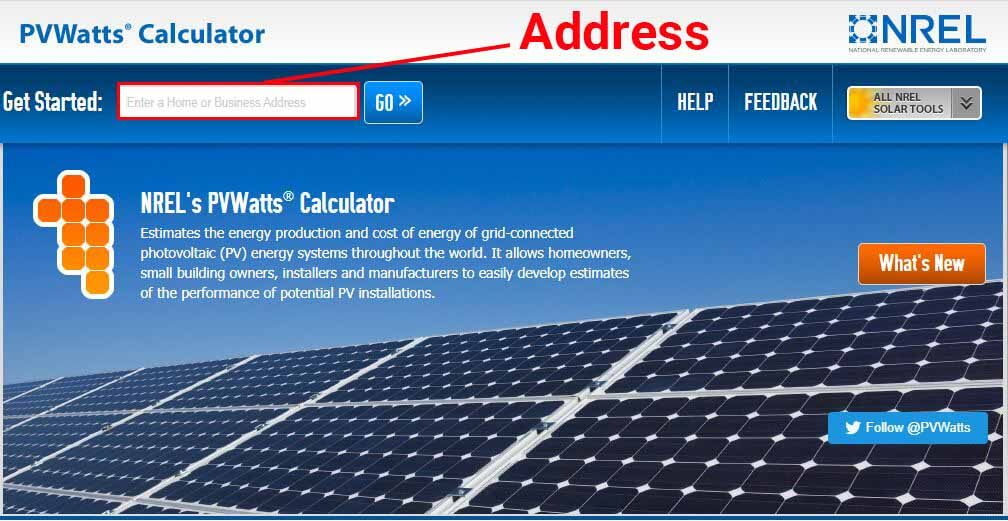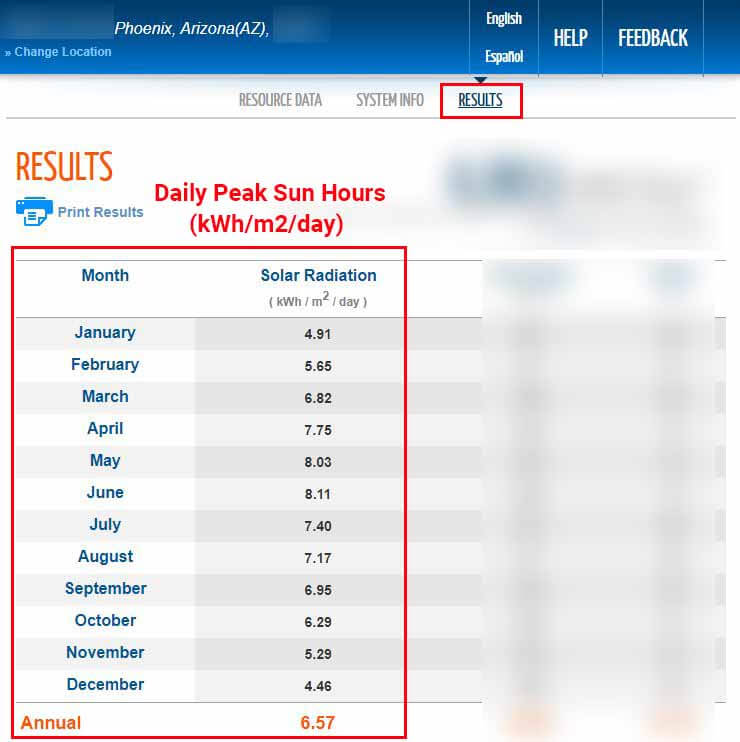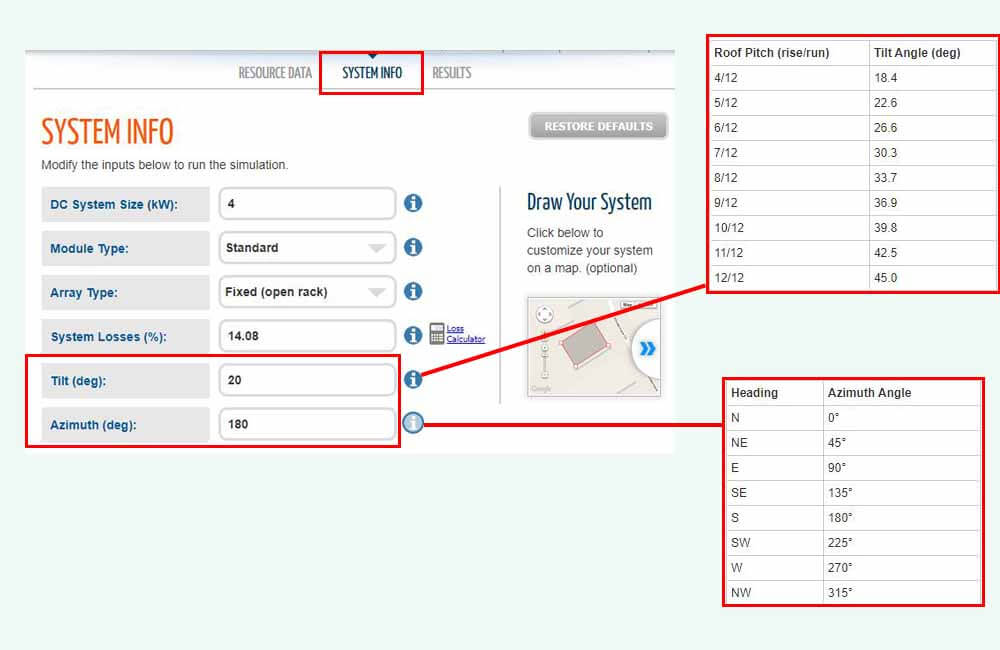# How many solar panels to run 3 ton air conditioner?

3 ton air conditioners consume a substantial amount of energy, yet it is still possible to offset that amount of energy consumption with solar. But the question remains, how much solar do you need?

The amount of solar power or number of solar panels needed to run a 3 ton air conditioner will depend on 2 variables:

• The amount of energy that your 3 ton air conditioner consumes on a daily basis.
• The amount of sunlight that is available to you.

Once you determine these 2 variables, the rest is simple calculations.

In this article, I’ll show you how to determine these 2 variables, and how to use them to calculate the amount of solar power that you need to run your 3 ton AC unit.

## How many solar panels to run 3 ton air conditioner?

In air conditioning, 3 tons is equivalent to 36000 BTUs, and air conditioners of this size consume about 2000 to 3000 Watt-hours per hour of run time (2-3 kWh/hour), depending on things like temperature, insulation, and age of the unit.

As a rule of thumb, you would need around 3000 Watts (3kW) of solar panels to run a 3-ton air conditioner for 8 hours a day. A solar system of this size would consist of 8 to 12 residential solar panels.

The following table estimates the energy consumption of 3-ton air conditioners based on their run time, and the amount of solar power needed to run them:

As mentioned above, a more accurate estimate can be calculated using the average daily energy consumption of the 3 ton AC unit, and the average amount of sunlight that your solar panels would receive on a daily basis (Peak Sun Hours).

This can be expressed via the following formula:

Solar Power Needed (Watts) = Air conditioner’s daily energy consumption (Watt-hours) ÷ Daily Peak Sun Hours

First, let’s see how you can determine the energy usage of your air conditioner.

### How much energy does a 3 ton air conditioner use?

The energy consumption of an air conditioner mainly depends on these factors:

• The efficiency of the air conditioner: represented by an Energy Efficiency Ratio (EER, SEER, CEER)
• Temperature: the outdoor temperature and the indoor temperature setpoint
• Insulation: the quality of the overall insulation of your home and the condition of the ductwork

Even if you know the capacity of the AC unit (3 tons or 36000 BTUs in this case), these factors make it a little hard to estimate its energy consumption. The most precise way to determine this energy consumption is to measure it using electricity monitoring devices such as the Kill-A-Watt meter or the Emporia monitor.

However, in my article about the electricity usage of air conditioners, I provide a simple rule of thumb that you can take advantage of to get some rough estimates:

Air Conditioner’s Energy Usage (Watt-hours) = Power Rating (Watts) x Run Time (hours) x 0.75

For example, consider a 3 ton air conditioner that has a wattage rating of 3300 Watts, and that is usually left on for 8 hours a day. The energy consumption of this AC unit can be estimated as such:

Air Conditioner’s Energy Usage (Watt-hours) = Power Rating (Watts) x Run Time (hours) x 0.75

Air Conditioner’s Energy Usage (Watt-hours) =3300 Watts x 8 hours x 0.75

Air Conditioner’s Energy Usage (Watt-hours) =19800 Watt-hours

Air Conditioner’s Energy Usage (kiloWatt-hours) =19.8 kWh

If you don’t know the power rating (watts) of your 3 ton AC unit, or if you have some confusion around the difference between watts (power) and watt-hours (energy), feel free to read up more on the topic here: How many watts does an air conditioner use?

For simplicity, I’ll use 19.8 kWh as the daily energy consumption for the rest of this article.

Once you have an estimate or an accurate measurement, the next step would be to determine the average amount of sunlight (Peak Sun Hours) that is available to you on a daily basis.

### How much sunlight do you get?

The power output of a solar panel depends directly on the amount of sunlight that it receives, which is measured in W/m2 (Watts per Square Meter) and referred to as Solar Irradiance. And solar panels need exactly 1000W/m2 of sunlight to produce 100% of their rated power.

For example, a 100W solar panel can only produce 100 Watts of power at a given moment if the Solar Irradiance is 1000W/m2 at that given moment. If the same solar panel only receives 800W/m2 of sunlight, it’ll only generate 80 Watts.

But what we’re looking for is energy production, not power production.

Thankfully, we can also measure the amount of energy that a location receives from the sun over a certain period. This amount of energy is measured in kWh/m(kiloWatt-hours per Square meter) and is referred to as Peak Sun Hours. 1 Peak Sun Hour is equivalent to 1kWh/m2 (or 1000Wh/m2).

And Peak Sun Hours are useful in predicting the amount of energy that a solar system is capable of producing in a certain location:

Daily Energy Production (Watt-hours) = System Power Rating (Watts) x Daily Peak Sun Hours

For example, a location that – on average – receives 6kWh/m2 of energy from the sun on a daily basis, could be said to receive 6 Peak Sun Hours per day. If we install 1000 Watts of solar in this particular location, the system would – on average – generate 6000 Watt-hours of energy per day:

Daily Energy Production (Watt-hours) = System Power Rating (Watts) x Daily Peak Sun Hours

Daily Energy Production (Watt-hours) = 1000 Watts x 6 Peak Sun Hours

Daily Energy Production (Watt-hours) = 6000 Watt-hours

So logically, if you know the amount of energy that you need the solar panels to produce, and the Peak Sun Hours that these solar panels would get, you can calculate the Power Rating of the system that you need:

System Power Rating (Watts) = Air conditioner’s daily energy consumption (Watt-hours) ÷ Daily Peak Sun Hours

So, how many Peak Sun Hours do you get per day?

You can easily determine your Peak Sun Hours by using the PVWatts Calculator provided by NREL (The National Renewable Energy Laboratory). Based on your address, the PVWatts Calculator can estimate the average daily Peak Sun Hours that you get (annual and monthly averages).For example, I submitted a random Phoenix, AZ address, and in the Results section, the tool provided the following:According to the tool, the annual average daily Peak Sun Hours that this location receives is 6.57 (6.57kWh/m2/day). The calculator also provides monthly averages, which is pretty useful.

It is also important to note that the calculator assumes that your solar panels will be facing south and installed on a surface with a 20-degree tilt angle (4-5/12 roof pitch). If this is not the case, you can change these variables in the System Info section of the tool:Different tilt angles and Azimuth values will result in different Peak Sun Hour values.

### Example:

In this example, I’ll assume that our 3 ton air conditioner consumes 19.8 kWh of energy per day on average and that we get 6.57 Peak Sun Hours per day. Based on these values, the amount of solar power that we need can be calculated as such:

Solar Power Needed (Watts) = Air conditioner’s daily energy consumption (Watt-hours) ÷ Daily Peak Sun Hours

Solar Power Needed (Watts) = 19800 Watt-hours ÷ 6.57 Peak Sun Hours

Solar Power Needed (Watts) = 3013.7 Watts

So, to run our 3 ton AC unit, we would need 3013.7 Watts of solar power. To account for system losses, cloudy days, and extreme temperatures, a good rule of thumb would be to bump this number up to 3500 Watts.

Depending on which solar panels you use, a 3.5kW solar system would generally consist of 10 to 14 residential solar panels.

Now, it is also important to note that if you’re trying to build an off-grid system that can run your 3 ton AC unit, you will also need the following components:

• A battery bank
• A solar charge controller
• An inverter

If this is indeed the case, please refer to the following pages:

Step 1 – Size your battery bank: How many 12 Volt batteries to run an air conditioner on solar?

Step 2 – Size your solar charge controller: MPPT solar charge controller calculator

Step 3 – Size the inverter: What size inverter do you need for your air conditioner?